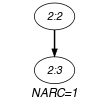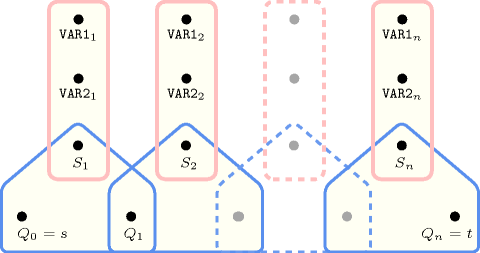## 5.227. lex_different

Origin

Used for defining $\mathrm{𝚕𝚎𝚡}_\mathrm{𝚊𝚕𝚕𝚍𝚒𝚏𝚏𝚎𝚛𝚎𝚗𝚝}$.

Constraint

$\mathrm{𝚕𝚎𝚡}_\mathrm{𝚍𝚒𝚏𝚏𝚎𝚛𝚎𝚗𝚝}\left(\mathrm{𝚅𝙴𝙲𝚃𝙾𝚁}\mathtt{1},\mathrm{𝚅𝙴𝙲𝚃𝙾𝚁}\mathtt{2}\right)$

Synonyms

$\mathrm{𝚍𝚒𝚏𝚏𝚎𝚛𝚎𝚗𝚝}$, $\mathrm{𝚍𝚒𝚏𝚏}$.

Arguments
 $\mathrm{𝚅𝙴𝙲𝚃𝙾𝚁}\mathtt{1}$ $\mathrm{𝚌𝚘𝚕𝚕𝚎𝚌𝚝𝚒𝚘𝚗}\left(\mathrm{𝚟𝚊𝚛}-\mathrm{𝚍𝚟𝚊𝚛}\right)$ $\mathrm{𝚅𝙴𝙲𝚃𝙾𝚁}\mathtt{2}$ $\mathrm{𝚌𝚘𝚕𝚕𝚎𝚌𝚝𝚒𝚘𝚗}\left(\mathrm{𝚟𝚊𝚛}-\mathrm{𝚍𝚟𝚊𝚛}\right)$
Restrictions
 $\mathrm{𝚛𝚎𝚚𝚞𝚒𝚛𝚎𝚍}$$\left(\mathrm{𝚅𝙴𝙲𝚃𝙾𝚁}\mathtt{1},\mathrm{𝚟𝚊𝚛}\right)$ $\mathrm{𝚛𝚎𝚚𝚞𝚒𝚛𝚎𝚍}$$\left(\mathrm{𝚅𝙴𝙲𝚃𝙾𝚁}\mathtt{2},\mathrm{𝚟𝚊𝚛}\right)$ $|\mathrm{𝚅𝙴𝙲𝚃𝙾𝚁}\mathtt{1}|>0$ $|\mathrm{𝚅𝙴𝙲𝚃𝙾𝚁}\mathtt{1}|=|\mathrm{𝚅𝙴𝙲𝚃𝙾𝚁}\mathtt{2}|$
Purpose

Vectors $\mathrm{𝚅𝙴𝙲𝚃𝙾𝚁}\mathtt{1}$ and $\mathrm{𝚅𝙴𝙲𝚃𝙾𝚁}\mathtt{2}$ differ in at least one component.

Example
$\left(〈5,2,7,1〉,〈5,3,7,1〉\right)$

The $\mathrm{𝚕𝚎𝚡}_\mathrm{𝚍𝚒𝚏𝚏𝚎𝚛𝚎𝚗𝚝}$ constraint holds since $\mathrm{𝚅𝙴𝙲𝚃𝙾𝚁}\mathtt{1}=$ $〈5,2,7,1〉$ and $\mathrm{𝚅𝙴𝙲𝚃𝙾𝚁}\mathtt{2}=$ $〈5,3,7,1〉$ differ in their second component.

Typical
 $|\mathrm{𝚅𝙴𝙲𝚃𝙾𝚁}\mathtt{1}|>1$ $\mathrm{𝚛𝚊𝚗𝚐𝚎}$$\left(\mathrm{𝚅𝙴𝙲𝚃𝙾𝚁}\mathtt{1}.\mathrm{𝚟𝚊𝚛}\right)>1$ $\mathrm{𝚛𝚊𝚗𝚐𝚎}$$\left(\mathrm{𝚅𝙴𝙲𝚃𝙾𝚁}\mathtt{2}.\mathrm{𝚟𝚊𝚛}\right)>1$
Symmetries
• Arguments are permutable w.r.t. permutation $\left(\mathrm{𝚅𝙴𝙲𝚃𝙾𝚁}\mathtt{1},\mathrm{𝚅𝙴𝙲𝚃𝙾𝚁}\mathtt{2}\right)$.

• Items of $\mathrm{𝚅𝙴𝙲𝚃𝙾𝚁}\mathtt{1}$ and $\mathrm{𝚅𝙴𝙲𝚃𝙾𝚁}\mathtt{2}$ are permutable (same permutation used).

Arg. properties

Extensible wrt. $\mathrm{𝚅𝙴𝙲𝚃𝙾𝚁}\mathtt{1}$ and $\mathrm{𝚅𝙴𝙲𝚃𝙾𝚁}\mathtt{2}$ (add items at same position).

Reformulation

The $\mathrm{𝚕𝚎𝚡}_\mathrm{𝚍𝚒𝚏𝚏𝚎𝚛𝚎𝚗𝚝}$$\left(〈\mathrm{𝚟𝚊𝚛}-{U}_{1},\mathrm{𝚟𝚊𝚛}-{U}_{2},\cdots ,\mathrm{𝚟𝚊𝚛}-{U}_{|\mathrm{𝚅𝙴𝙲𝚃𝙾𝚁}\mathtt{1}|}〉,〈\mathrm{𝚟𝚊𝚛}-{V}_{1},\mathrm{𝚟𝚊𝚛}-{V}_{2},\cdots ,\mathrm{𝚟𝚊𝚛}-{V}_{|\mathrm{𝚅𝙴𝙲𝚃𝙾𝚁}\mathtt{2}|}〉\right)$ constraint can be expressed in term of the following disjunction of disequality constraints ${U}_{1}\ne {V}_{1}\vee {U}_{2}\ne {V}_{2}\vee \cdots \vee {U}_{|\mathrm{𝚅𝙴𝙲𝚃𝙾𝚁}\mathtt{1}|}\ne {V}_{|\mathrm{𝚅𝙴𝙲𝚃𝙾𝚁}\mathtt{2}|}$.

Used in
Keywords
Arc input(s)

$\mathrm{𝚅𝙴𝙲𝚃𝙾𝚁}\mathtt{1}$ $\mathrm{𝚅𝙴𝙲𝚃𝙾𝚁}\mathtt{2}$

Arc generator
$\mathrm{𝑃𝑅𝑂𝐷𝑈𝐶𝑇}$$\left(=\right)↦\mathrm{𝚌𝚘𝚕𝚕𝚎𝚌𝚝𝚒𝚘𝚗}\left(\mathrm{𝚟𝚎𝚌𝚝𝚘𝚛}\mathtt{1},\mathrm{𝚟𝚎𝚌𝚝𝚘𝚛}\mathtt{2}\right)$

Arc arity
Arc constraint(s)
$\mathrm{𝚟𝚎𝚌𝚝𝚘𝚛}\mathtt{1}.\mathrm{𝚟𝚊𝚛}\ne \mathrm{𝚟𝚎𝚌𝚝𝚘𝚛}\mathtt{2}.\mathrm{𝚟𝚊𝚛}$
Graph property(ies)
$\mathrm{𝐍𝐀𝐑𝐂}$$\ge 1$

Graph model

Parts (A) and (B) of Figure 5.227.1 respectively show the initial and final graph associated with the Example slot. Since we use the $\mathrm{𝐍𝐀𝐑𝐂}$ graph property, the unique arc of the final graph is stressed in bold. It corresponds to a component where the two vectors differ.

##### Figure 5.227.1. Initial and final graph of the $\mathrm{𝚕𝚎𝚡}_\mathrm{𝚍𝚒𝚏𝚏𝚎𝚛𝚎𝚗𝚝}$ constraint(a) (b)
Automaton

Figure 5.227.2 depicts the automaton associated with the $\mathrm{𝚕𝚎𝚡}_\mathrm{𝚍𝚒𝚏𝚏𝚎𝚛𝚎𝚗𝚝}$ constraint. Let $\mathrm{𝚅𝙰𝚁}{\mathtt{1}}_{i}$ and $\mathrm{𝚅𝙰𝚁}{\mathtt{2}}_{i}$ respectively be the $\mathrm{𝚟𝚊𝚛}$ attributes of the ${i}^{th}$ items of the $\mathrm{𝚅𝙴𝙲𝚃𝙾𝚁}\mathtt{1}$ and the $\mathrm{𝚅𝙴𝙲𝚃𝙾𝚁}\mathtt{2}$ collections. To each pair $\left(\mathrm{𝚅𝙰𝚁}{\mathtt{1}}_{i},\mathrm{𝚅𝙰𝚁}{\mathtt{2}}_{i}\right)$ corresponds a 0-1 signature variable ${S}_{i}$ as well as the following signature constraint: $\mathrm{𝚅𝙰𝚁}{\mathtt{1}}_{i}=\mathrm{𝚅𝙰𝚁}{\mathtt{2}}_{i}⇔{S}_{i}$.

##### Figure 5.227.2. Automaton of the $\mathrm{𝚕𝚎𝚡}_\mathrm{𝚍𝚒𝚏𝚏𝚎𝚛𝚎𝚗𝚝}$ constraint##### Figure 5.227.3. Hypergraph of the reformulation corresponding to the automaton of the $\mathrm{𝚕𝚎𝚡}_\mathrm{𝚍𝚒𝚏𝚏𝚎𝚛𝚎𝚗𝚝}$ constraint